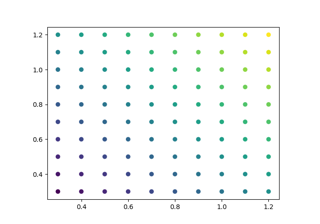# matplotlib.axes.Axes.set_xmargin#

Axes.set_xmargin(m)[source]#

Set padding of X data limits prior to autoscaling.

m times the data interval will be added to each end of that interval before it is used in autoscaling. For example, if your data is in the range [0, 2], a factor of `m = 0.1` will result in a range [-0.2, 2.2].

Negative values -0.5 < m < 0 will result in clipping of the data range. I.e. for a data range [0, 2], a factor of `m = -0.1` will result in a range [0.2, 1.8].

Parameters
mfloat greater than -0.5

## Examples using `matplotlib.axes.Axes.set_xmargin`#Automatically setting tick positions

Automatically setting tick positions